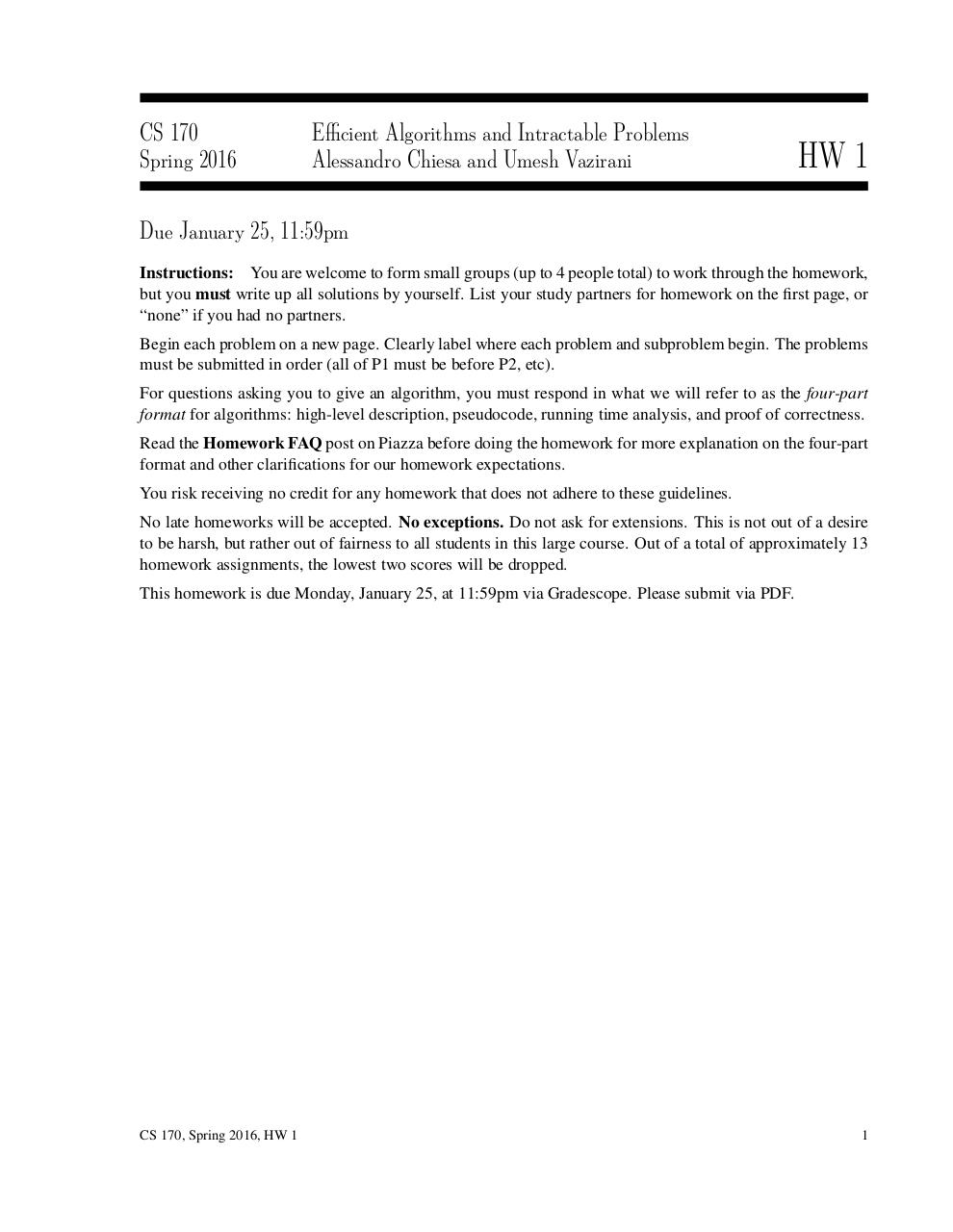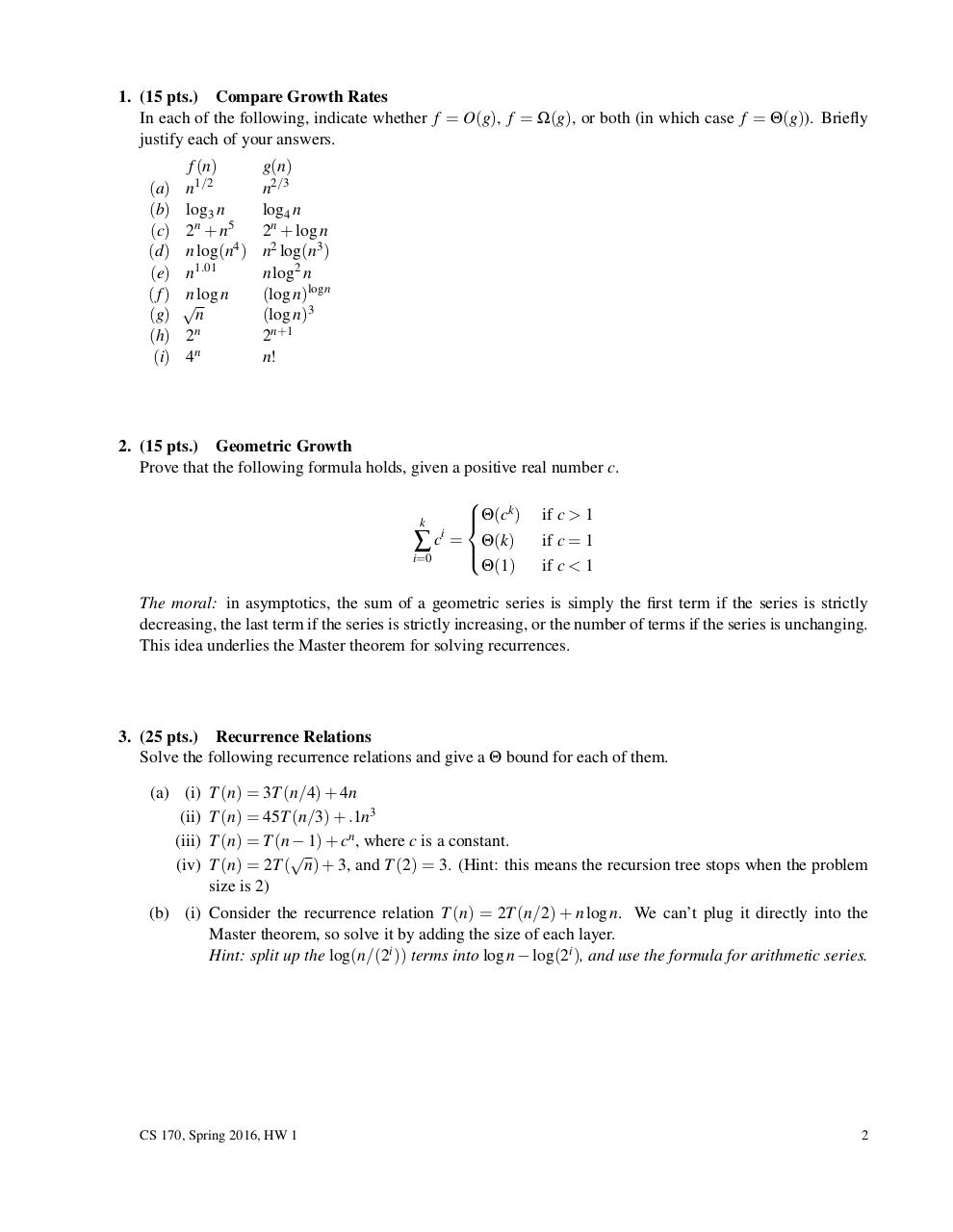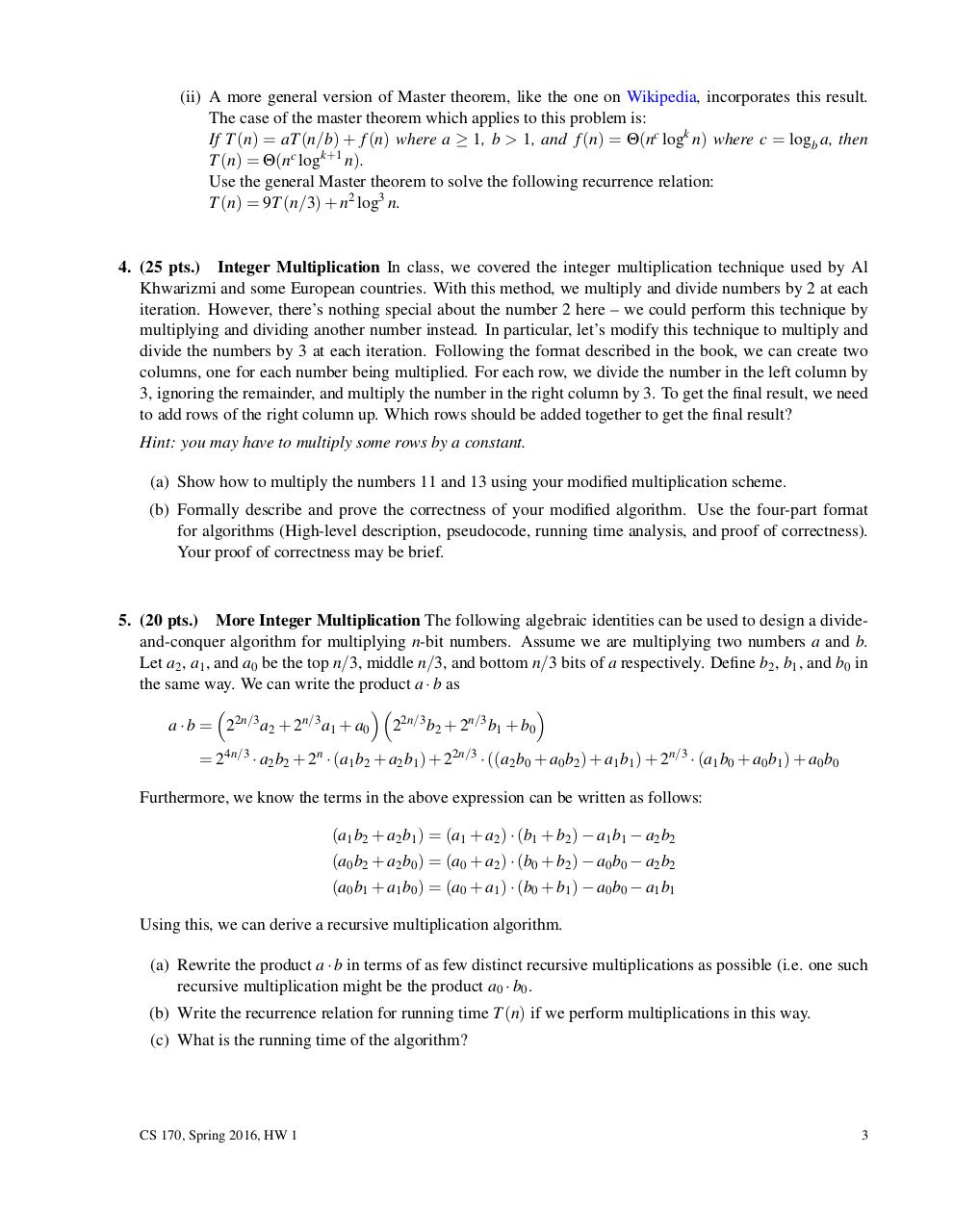# hw1v3 .pdf

### File information

Original filename: hw1v3.pdf

This PDF 1.5 document has been generated by LaTeX with hyperref package / pdfTeX-1.40.16, and has been sent on pdf-archive.com on 20/01/2016 at 08:51, from IP address 50.161.x.x. The current document download page has been viewed 895 times.
File size: 97 KB (3 pages).
Privacy: public file

hw1v3.pdf (PDF, 97 KB)

### Document preview

CS 170
Spring 2016

Efficient Algorithms and Intractable Problems
Alessandro Chiesa and Umesh Vazirani

HW 1

Due January 25, 11:59pm
Instructions: You are welcome to form small groups (up to 4 people total) to work through the homework,
but you must write up all solutions by yourself. List your study partners for homework on the first page, or
“none” if you had no partners.
Begin each problem on a new page. Clearly label where each problem and subproblem begin. The problems
must be submitted in order (all of P1 must be before P2, etc).
For questions asking you to give an algorithm, you must respond in what we will refer to as the four-part
format for algorithms: high-level description, pseudocode, running time analysis, and proof of correctness.
Read the Homework FAQ post on Piazza before doing the homework for more explanation on the four-part
format and other clarifications for our homework expectations.
You risk receiving no credit for any homework that does not adhere to these guidelines.
No late homeworks will be accepted. No exceptions. Do not ask for extensions. This is not out of a desire
to be harsh, but rather out of fairness to all students in this large course. Out of a total of approximately 13
homework assignments, the lowest two scores will be dropped.
This homework is due Monday, January 25, at 11:59pm via Gradescope. Please submit via PDF.

CS 170, Spring 2016, HW 1

1

1. (15 pts.) Compare Growth Rates
In each of the following, indicate whether f = O(g), f = Ω(g), or both (in which case f = Θ(g)). Briefly
(a)
(b)
(c)
(d)
(e)
(f)
(g)
(h)
(i)

f (n)
n1/2
log3 n
2n + n5
n log(n4 )
n1.01
n log n

n
2n
4n

g(n)
n2/3
log4 n
2n + log n
n2 log(n3 )
n log2 n
(log n)log n
(log n)3
2n+1
n!

2. (15 pts.) Geometric Growth
Prove that the following formula holds, given a positive real number c.

k

Θ(c )
∑ ci = Θ(k)

i=0
Θ(1)
k

if c &gt; 1
if c = 1
if c &lt; 1

The moral: in asymptotics, the sum of a geometric series is simply the first term if the series is strictly
decreasing, the last term if the series is strictly increasing, or the number of terms if the series is unchanging.
This idea underlies the Master theorem for solving recurrences.

3. (25 pts.) Recurrence Relations
Solve the following recurrence relations and give a Θ bound for each of them.
(a) (i)
(ii)
(iii)
(iv)

T (n) = 3T (n/4) + 4n
T (n) = 45T (n/3) + .1n3
T (n) = T (n − 1) + cn , where c is a constant.

T (n) = 2T ( n) + 3, and T (2) = 3. (Hint: this means the recursion tree stops when the problem
size is 2)

(b) (i) Consider the recurrence relation T (n) = 2T (n/2) + n log n. We can’t plug it directly into the
Master theorem, so solve it by adding the size of each layer.
Hint: split up the log(n/(2i )) terms into log n − log(2i ), and use the formula for arithmetic series.

CS 170, Spring 2016, HW 1

2

(ii) A more general version of Master theorem, like the one on Wikipedia, incorporates this result.
The case of the master theorem which applies to this problem is:
If T (n) = aT (n/b) + f (n) where a ≥ 1, b &gt; 1, and f (n) = Θ(nc logk n) where c = logb a, then
T (n) = Θ(nc logk+1 n).
Use the general Master theorem to solve the following recurrence relation:
T (n) = 9T (n/3) + n2 log3 n.

4. (25 pts.) Integer Multiplication In class, we covered the integer multiplication technique used by Al
Khwarizmi and some European countries. With this method, we multiply and divide numbers by 2 at each
iteration. However, there’s nothing special about the number 2 here – we could perform this technique by
multiplying and dividing another number instead. In particular, let’s modify this technique to multiply and
divide the numbers by 3 at each iteration. Following the format described in the book, we can create two
columns, one for each number being multiplied. For each row, we divide the number in the left column by
3, ignoring the remainder, and multiply the number in the right column by 3. To get the final result, we need
to add rows of the right column up. Which rows should be added together to get the final result?
Hint: you may have to multiply some rows by a constant.
(a) Show how to multiply the numbers 11 and 13 using your modified multiplication scheme.
(b) Formally describe and prove the correctness of your modified algorithm. Use the four-part format
for algorithms (High-level description, pseudocode, running time analysis, and proof of correctness).
Your proof of correctness may be brief.

5. (20 pts.) More Integer Multiplication The following algebraic identities can be used to design a divideand-conquer algorithm for multiplying n-bit numbers. Assume we are multiplying two numbers a and b.
Let a2 , a1 , and a0 be the top n/3, middle n/3, and bottom n/3 bits of a respectively. Define b2 , b1 , and b0 in
the same way. We can write the product a · b as



a · b = 22n/3 a2 + 2n/3 a1 + a0 22n/3 b2 + 2n/3 b1 + b0
= 24n/3 · a2 b2 + 2n · (a1 b2 + a2 b1 ) + 22n/3 · ((a2 b0 + a0 b2 ) + a1 b1 ) + 2n/3 · (a1 b0 + a0 b1 ) + a0 b0
Furthermore, we know the terms in the above expression can be written as follows:
(a1 b2 + a2 b1 ) = (a1 + a2 ) · (b1 + b2 ) − a1 b1 − a2 b2
(a0 b2 + a2 b0 ) = (a0 + a2 ) · (b0 + b2 ) − a0 b0 − a2 b2
(a0 b1 + a1 b0 ) = (a0 + a1 ) · (b0 + b1 ) − a0 b0 − a1 b1
Using this, we can derive a recursive multiplication algorithm.
(a) Rewrite the product a · b in terms of as few distinct recursive multiplications as possible (i.e. one such
recursive multiplication might be the product a0 · b0 .
(b) Write the recurrence relation for running time T (n) if we perform multiplications in this way.
(c) What is the running time of the algorithm?

CS 170, Spring 2016, HW 1

3#### HTML Code

Copy the following HTML code to share your document on a Website or Blog

#### QR Code### Related keywords